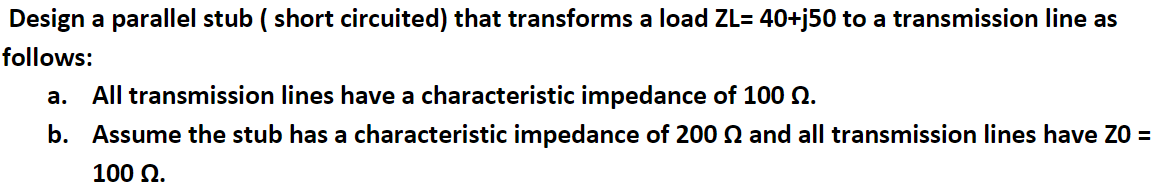# (Solved): Design a parallel stub ( short circuited) that transforms a load ZL=40+j50 to a transmission line a ...Design a parallel stub ( short circuited) that transforms a load to a transmission line as follows: a. All transmission lines have a characteristic impedance of . b. Assume the stub has a characteristic impedance of and all transmission lines have .

We have an Answer from Expert